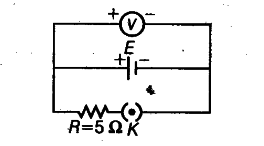# Write any two factors on which internal resistance of a cell depends

Write any two factors on which internal
resistance of a cell depends. The reading on a high resistance voltmeter, when a cell is connected across it, is 2.2 V. When the terminals of the cell are also connected to a resistance of 5 Ω as shown in the circuit, the voltmeter reading drops to 1.8 V. Find the internal resistance of the cell.The high resistance voltmeter means, that current will flow through it. Hence, there is no potential difference across it. So, the reading shown by the high resistance voltmeter can be taken as the emf of the cell.
The internal resistance of a cell depends on
(i) the concentration of electrolyte and
(ii) distance between the two electrodes.
The emf of cell, E = 2.2 V .
The terminal voltage across cell when 5 Ω resistance R is connected across it, V= 1.8 V
Let internal resistance = r
Internal resistance, r= r(E/V -1)
= 5(2.2/1.8-1) = 5 x 0.4/1.8 = 2/1.8 =10/9 Ω